# Types of Resistors

Want create site? Find Free WordPress Themes and plugins.

Resistors can be classified into different types according to their construction.

Wire-wound resistors are made by wrapping high-resistance wire around an insulated cylinder, as illustrated in Figure 1. This type of resistor is generally used in circuits that carry high currents. Large wire-wound resistors are called power resistors and range in size from ½ watt to tens or even hundreds of watts.Figure 1 Wire-wound resistor.

Special wire-wound fusible resistors (Figure 2) are designed to burn open when their power rating is exceeded. They serve the dual functions of a fuse and resistor to limit the current. To prevent flames or explosion when fusing, the device has an inflammable construction with high dielectric strength.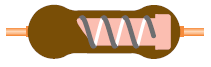Figure 2 Fusible resistor.

Carbon-composition resistors are made from a paste consisting of carbon graphite and a resin bonding material, as illustrated in Figure 3.

The resistance of a carbon resistor is determined by the amount of carbon graphite used in making the resistor. The resistor element is enclosed in a plastic case for insulation and mechanical strength. Joined to the two ends of the carbon resistance element are metal caps with leads for soldering the connections into a circuit.

Carbon-composition resistors at one time were the most common type used. Generally, they cannot handle large currents, and their actual value of resistance can vary as much as 20 percent from their rated value.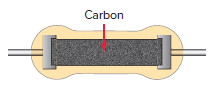Figure 3 Carbon-composition resistor.

Presently, the most popular type of resistor is the film-type resistor shown in Figure 4. In these resistors, a resistance film is deposited on a non-conductive rod. Then the value of resistance is set by cutting a spiral groove through the film. The length and width of the groove determine the resistance value. This method of manufacture allows for much closer tolerance resistors (1 percent or less) as compared to the simpler carbon-composition types.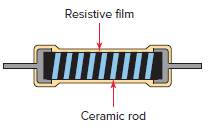Figure 4 Film-type resistor.

chip resistor is a surface-mounted printed circuit board mini-resistor. Chip resistors differ from conventional axial lead resistors in that they are soldered directly onto the conductive tracks of printed circuit boards.

There are two basic configurations for chip resistors: single resistor and resistor chip array (Figure 5). Single-chip resistors are standard, passive resistors with a single resistance value. Resistor chip arrays contain several resistors in a single package.

The chip resistors shown are marked with a three-digit code (similar to the resistor color code). The first two numbers indicate the significant digits, and the third will be the multiplier, telling you the power of 10 to which the two significant digits must be multiplied.Figure 5 Chip resistors.

Resistors are also classified as being fixed, adjustable, or variable. The fixed resistor, shown in Figure 6, has a specified value that cannot be changed. The sliding contact adjustable resistor, shown in Figure 7, has an adjustable collar that can be moved to tap off any resistance within the ohmic value range of the resistor.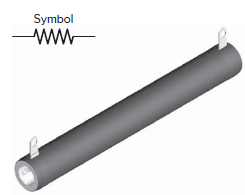Figure 6 Fixed resistor.The variable resistor, shown in Figure 8, is designed to provide continuous adjustment of resistance. Variable resistors have a resistive body and a wiper. The wiper slides on the resistive body, changing the length of the resistive material between one end of the device and the wiper.

Since resistance depends directly on length, increasing the length of the resistive material between the end of the resistor and the wiper makes the resistance higher.Figure 8 Variable resistor.

## Rheostats and Potentiometers

Variable resistors are of two different types: rheostat and potentiometer. A rheostat is a variable resistor connected using only two of its terminals. The rheostat is used to control current by varying the resistance in a circuit.

Figure 8 shows a rheostat in a low-power lamp dimmer circuit. By changing the resistance of the rheostat, the current flow through it is varied.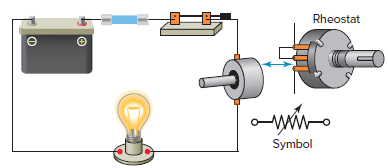Figure 8 Rheostat lamp dimmer circuit.

potentiometer (or pot, as it is commonly known) is a variable resistor that makes use of all three of its terminals. Potentiometers are generally used to adjust the level of AC or DC voltage.

Figure 9 shows a variable DC voltage control potentiometer circuit. The two fixed maximum-resistance leads are connected across the voltage source, and the variable wiper arm lead provides a voltage that varies from 0- to the 9-V maximum.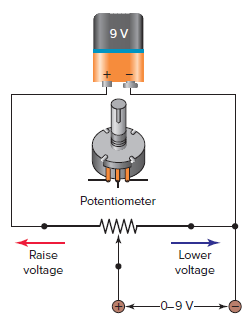Figure 9 Voltage control potentiometer circuit.

Trimmer (trim) potentiometers are used when the ohmic value of a resistor is set at the time a circuit is manufactured and tested. They are generally a miniature size and mounted on a printed circuit board. Often they are used to fine-tune or calibrate a circuit.

Unlike a typical potentiometer that rotates just short of one complete revolution, some trim pots, such as the one shown in Figure 10, are what we call multiturn pots. A 10-turn trim pot, for example, must be rotated completely 10 times in order for the wiper to move from one end of the resistive element to the other. This allows for very precise adjustments.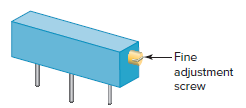Figure 10 Multiturn trimmer potentiometer.

Whether rheostat or potentiometer, the resistive track can be classified as either a linear or a tapered (nonlinear) resistance. With a linear rheostat or potentiometer the resistance changes in direct proportion to rotation. The resistance for a nonlinear rheostat or potentiometer changes more gradually at one end, with bigger changes at the opposite end. The effect is accomplished by different densities of the resistance element in one-half than in the other.

Audio volume controls are of the nonlinear type, allowing greater control of loudness at normal or low listening levels.

Review Question

1. State two functions served by a fusible resistor.
2. What advantage do film resistors have over carbon-composition types?
3. What are the two configurations for chip resistors?
4. In what way is the construction of an adjustable resistor different from that of a fixed resistor?
5. Compare the connection and control function of a rheostat with that of a potentiometer.

1. They serve the dual functions of a fuse and resistor to limit the current.
2. They cost less and have more precise resistance values.
3. There are two basic configurations for chip resistors: single resistor and resistor chip arrays.
4. A variable resistor has to have a mechanical connection that changes the position of the connection so the resistance of the component changes.
5. A rheostat is a variable resistor connected using only two of its terminals. The rheostat is used to control current by varying the resistance in a circuit. A potentiometer is a variable resistor that makes use of all three of its terminals. Potentiometers are generally used to adjust voltage.